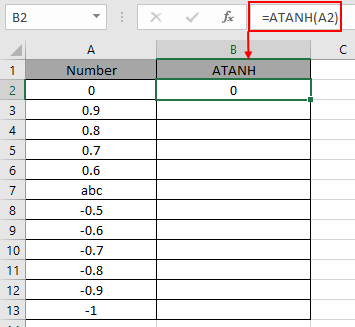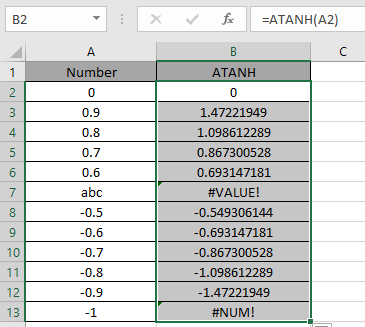# How to use the ATANH Function in Excel

TANH function is a Mathematical function to calculate the hyperbolic Tangent of the value. TANH function is built in excel function. Hyperbolic function of TANH defined using the exponential function.

TANH (x) = SINH (x) / COSH (x)

TANH (x) = (ex- e-x) / (ex+ e-x)

Where ex = exponential function, e = 2.71828

So, it means Tangent hyperbolic function returns the value as the formula stated above. Now if we wish to return the inverse of Tangent hyperbolic function, excel ATANH function does our work. The below stated

x =  TANH -1(ex- e-x) / (ex+ e-x)    { in mathematics }

x =  ATANH [(ex- e-x) / (ex+ e-x)]   { in Excel }

So, The inverse hyperbolic Tangent is the value whose hyperbolic Tangent is the number, so ATANH(TANH(number)) equals number.

ATANH ( TANH ( number ) ) = number

ATANH function in excel returns inverse of the Tangent hyperbolic function in excel. The

Syntax:

= ATANH ( number )

number : input any real number between -1 and +1 (excluding -1 & +1).

Let’s understand this function using it in an example.Here we have values which will be input to the function and we need to find the inverse of the hyperbolic Tangent value using the ATANH function in excel

Use the formula:

=TANH ( A2 )

A2 : number given as cell reference.Here the argument to the function is provided as cell reference. As shown in the above snapshot, the Resultant comes out to be 0.

The value will calculated using the mathematical formulation stated :

x = TANH -1 (e0- e0) / (e0+ e0) = TANH -1( 0 ) = 0
Now copy the formula to other cells using the Ctrl + D shortcut key.Excel calculates the inverse of the Hyperbolic Tangent of a number using the TANH function.

Here are some of the observations gathered using the ATANH function

Note:

1. The function returns #VALUE! Error if the argument to the function is non - numeric.
2. The function returns #NUM error,
1. if the argument number is less than or equal to 1.
2. If the argument number is greater than or equal to 1.
3. Argument to the function can be provided directly or using the cell reference in excel.

As you can see, we can get the inverse of the Tangent hyperbolic function using ATANH function.

Hope you understood How to use ATANH function in Excel. Explore more articles on Mathematical function like ASINH function and ACOSH function here. Please state your query in the comment box below.

Related Articles

How to use the TANH function in Excel

How to use the TAN function in Excel

How to use Wildcards in Excel

Popular Articles

Edit a dropdown list

If with conditional formatting

If with wildcards

Vlookup by date

Terms and Conditions of use

The applications/code on this site are distributed as is and without warranties or liability. In no event shall the owner of the copyrights, or the authors of the applications/code be liable for any loss of profit, any problems or any damage resulting from the use or evaluation of the applications/code.